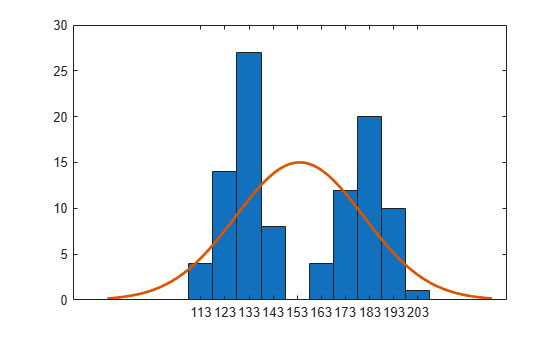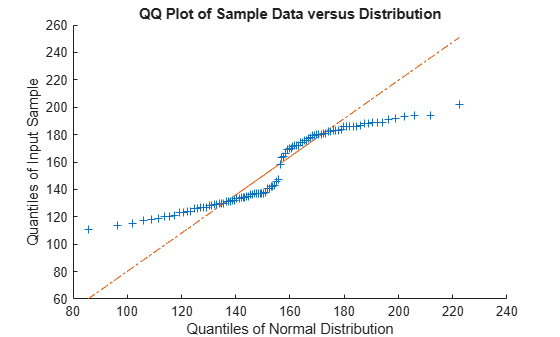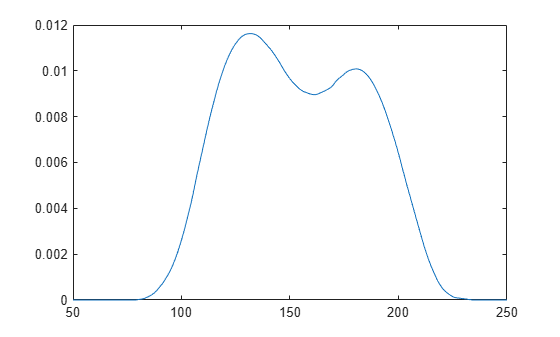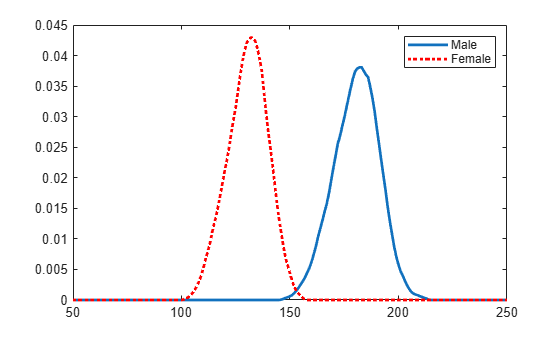# fitdist

Fit probability distribution object to data

## Syntax

``pd = fitdist(x,distname)``
``pd = fitdist(x,distname,Name,Value)``
``````[pdca,gn,gl] = fitdist(x,distname,'By',groupvar)``````
``````[pdca,gn,gl] = fitdist(x,distname,'By',groupvar,Name,Value)``````

## Description

example

````pd = fitdist(x,distname)` creates a probability distribution object by fitting the distribution specified by `distname` to the data in column vector `x`.```

example

````pd = fitdist(x,distname,Name,Value)` creates the probability distribution object with additional options specified by one or more name-value pair arguments. For example, you can indicate censored data or specify control parameters for the iterative fitting algorithm.```

example

``````[pdca,gn,gl] = fitdist(x,distname,'By',groupvar)``` creates probability distribution objects by fitting the distribution specified by `distname` to the data in `x` based on the grouping variable `groupvar`. It returns a cell array of fitted probability distribution objects, `pdca`, a cell array of group labels, `gn`, and a cell array of grouping variable levels, `gl`.```

example

``````[pdca,gn,gl] = fitdist(x,distname,'By',groupvar,Name,Value)``` returns the above output arguments using additional options specified by one or more name-value pair arguments. For example, you can indicate censored data or specify control parameters for the iterative fitting algorithm.```

## Examples

collapse all

Fit a normal distribution to sample data, and examine the fit by using a histogram and a quantile-quantile plot.

Load patient weights from the data file `patients.mat`.

```load patients x = Weight;```

Create a normal distribution object by fitting it to the data.

`pd = fitdist(x,'Normal')`
```pd = NormalDistribution Normal distribution mu = 154 [148.728, 159.272] sigma = 26.5714 [23.3299, 30.8674] ```

The distribution object display includes the parameter estimates for the mean (`mu`) and standard deviation (`sigma`), and the 95% confidence intervals for the parameters.

You can use the object functions of `pd` to evaluate the distribution and generate random numbers. Display the supported object functions.

`methods(pd)`
```Methods for class prob.NormalDistribution: cdf iqr negloglik plot std gather mean paramci proflik truncate icdf median pdf random var ```

For example, obtain the 95% confidence intervals by using the `paramci` function.

`ci95 = paramci(pd)`
```ci95 = 2×2 148.7277 23.3299 159.2723 30.8674 ```

Specify the significance level (`Alpha`) to obtain confidence intervals with a different confidence level. Compute the 99% confidence intervals.

`ci99 = paramci(pd,'Alpha',.01)`
```ci99 = 2×2 147.0213 22.4257 160.9787 32.4182 ```

Evaluate and plot the pdf values of the distribution.

```x_values = 50:1:250; y = pdf(pd,x_values); plot(x_values,y)```Create a histogram with the normal distribution fit by using the `histfit` function. `histfit` uses `fitdist` to fit a distribution to data.

`histfit(x)`The histogram shows that the data has two modes, and that the mode of the normal distribution fit is between those two modes.

Use `qqplot` to create a quantile-quantile plot of the quantiles of the sample data `x` versus the theoretical quantile values of the fitted distribution.

`qqplot(x,pd)`The plot is not a straight line, suggesting that the data does not follow a normal distribution.

Load patient weights from the data file `patients.mat`.

```load patients x = Weight;```

Create a kernel distribution object by fitting it to the data. Use the Epanechnikov kernel function.

`pd = fitdist(x,'Kernel','Kernel','epanechnikov')`
```pd = KernelDistribution Kernel = epanechnikov Bandwidth = 14.3792 Support = unbounded ```

Plot the pdf of the distribution.

```x_values = 50:1:250; y = pdf(pd,x_values); plot(x_values,y)```Load patient weights and genders from the data file `patients.mat`.

```load patients x = Weight;```

Create normal distribution objects by fitting them to the data, grouped by patient gender.

`[pdca,gn,gl] = fitdist(x,'Normal','By',Gender)`
```pdca=1×2 cell array {1x1 prob.NormalDistribution} {1x1 prob.NormalDistribution} ```
```gn = 2x1 cell {'Male' } {'Female'} ```
```gl = 2x1 cell {'Male' } {'Female'} ```

The cell array `pdca` contains two probability distribution objects, one for each gender group. The cell array `gn` contains two group labels. The cell array `gl` contains two group levels.

View each distribution in the cell array `pdca` to compare the mean, `mu`, and the standard deviation, `sigma`, grouped by patient gender.

`female = pdca{1} % Distribution for females`
```female = NormalDistribution Normal distribution mu = 180.532 [177.833, 183.231] sigma = 9.19322 [7.63933, 11.5466] ```
`male = pdca{2} % Distribution for males`
```male = NormalDistribution Normal distribution mu = 130.472 [128.183, 132.76] sigma = 8.30339 [6.96947, 10.2736] ```

Compute the pdf of each distribution.

```x_values = 50:1:250; femalepdf = pdf(female,x_values); malepdf = pdf(male,x_values);```

Plot the pdfs for a visual comparison of weight distribution by gender.

```figure plot(x_values,femalepdf,'LineWidth',2) hold on plot(x_values,malepdf,'Color','r','LineStyle',':','LineWidth',2) legend(gn,'Location','NorthEast') hold off```Load patient weights and genders from the data file `patients.mat`.

```load patients x = Weight;```

Create kernel distribution objects by fitting them to the data, grouped by patient gender. Use a triangular kernel function.

`[pdca,gn,gl] = fitdist(x,'Kernel','By',Gender,'Kernel','triangle');`

View each distribution in the cell array `pdca` to see the kernel distributions for each gender.

`female = pdca{1} % Distribution for females`
```female = KernelDistribution Kernel = triangle Bandwidth = 5.08961 Support = unbounded ```
`male = pdca{2} % Distribution for males`
```male = KernelDistribution Kernel = triangle Bandwidth = 4.25894 Support = unbounded ```

Compute the pdf of each distribution.

```x_values = 50:1:250; femalepdf = pdf(female,x_values); malepdf = pdf(male,x_values);```

Plot the pdfs for a visual comparison of weight distribution by gender.

```figure plot(x_values,femalepdf,'LineWidth',2) hold on plot(x_values,malepdf,'Color','r','LineStyle',':','LineWidth',2) legend(gn,'Location','NorthEast') hold off```## Input Arguments

collapse all

Input data, specified as a column vector. `fitdist` ignores `NaN` values in `x`. Additionally, any `NaN` values in the censoring vector or frequency vector cause `fitdist` to ignore the corresponding values in `x`.

Data Types: `double`

Distribution name, specified as one of the following character vectors or string scalars. The distribution specified by `distname` determines the type of the returned probability distribution object.

Distribution NameDescriptionDistribution Object
`'Beta'`Beta distribution`BetaDistribution`
`'Binomial'`Binomial distribution`BinomialDistribution`
`'BirnbaumSaunders'`Birnbaum-Saunders distribution`BirnbaumSaundersDistribution`
`'Burr'`Burr distribution`BurrDistribution`
`'Exponential'`Exponential distribution`ExponentialDistribution`
`'Extreme Value'` or `'ev'`Extreme Value distribution`ExtremeValueDistribution`
`'Gamma'`Gamma distribution`GammaDistribution`
`'Generalized Extreme Value'` or `'gev'`Generalized Extreme Value distribution`GeneralizedExtremeValueDistribution`
`'Generalized Pareto'` or `'gp'`Generalized Pareto distribution`GeneralizedParetoDistribution`
`'Half Normal'` or `'hn'`Half-normal distribution`HalfNormalDistribution`
`'InverseGaussian'`Inverse Gaussian distribution`InverseGaussianDistribution`
`'Kernel'`Kernel distribution`KernelDistribution`
`'Logistic'`Logistic distribution`LogisticDistribution`
`'Loglogistic'`Loglogistic distribution`LoglogisticDistribution`
`'Lognormal'`Lognormal distribution`LognormalDistribution`
`'Nakagami'`Nakagami distribution`NakagamiDistribution`
`'Negative Binomial'` or `'nbin'`Negative Binomial distribution`NegativeBinomialDistribution`
`'Normal'`Normal distribution`NormalDistribution`
`'Poisson'`Poisson distribution`PoissonDistribution`
`'Rayleigh'`Rayleigh distribution`RayleighDistribution`
`'Rician'`Rician distribution`RicianDistribution`
`'Stable'`Stable distribution`StableDistribution`
`'tLocationScale'`t Location-Scale distribution`tLocationScaleDistribution`
`'Weibull'` or `'wbl'`Weibull distribution`WeibullDistribution`

Grouping variable, specified as a categorical array, logical or numeric vector, character array, string array, or cell array of character vectors. Each unique value in a grouping variable defines a group.

For example, if `Gender` is a cell array of character vectors with values `'Male'` and `'Female'`, you can use `Gender` as a grouping variable to fit a distribution to your data by gender.

More than one grouping variable can be used by specifying a cell array of grouping variables. Observations are placed in the same group if they have common values of all specified grouping variables.

For example, if `Smoker` is a logical vector with values `0` for nonsmokers and `1` for smokers, then specifying the cell array `{Gender,Smoker}` divides observations into four groups: Male Smoker, Male Nonsmoker, Female Smoker, and Female Nonsmoker.

Example: `{Gender,Smoker}`

Data Types: `categorical` | `logical` | `single` | `double` | `char` | `string` | `cell`

### Name-Value Arguments

Specify optional pairs of arguments as `Name1=Value1,...,NameN=ValueN`, where `Name` is the argument name and `Value` is the corresponding value. Name-value arguments must appear after other arguments, but the order of the pairs does not matter.

Before R2021a, use commas to separate each name and value, and enclose `Name` in quotes.

Example: `fitdist(x,'Kernel','Kernel','triangle')` fits a kernel distribution object to the data in `x` using a triangular kernel function.

Logical flag for censored data, specified as a vector of logical values that is the same size as input vector `x`. The value is `1` when the corresponding element in `x` is a right-censored observation and `0` when the corresponding element is an exact observation. The default is a vector of `0`s, indicating that all observations are exact.

`fitdist` ignores any `NaN` values in this censoring vector. Additionally, any `NaN` values in `x` or the frequency vector cause `fitdist` to ignore the corresponding values in the censoring vector.

This argument is valid only if `distname` is `'BirnbaumSaunders'`, `'Burr'`, `'Exponential'`, `'ExtremeValue'`, `'Gamma'`, `'InverseGaussian'`, `'Kernel'`, `'Logistic'`, `'Loglogistic'`, `'Lognormal'`, `'Nakagami'`, `'Normal'`, `'Rician'`, `'tLocationScale'`, or `'Weibull'`.

Data Types: `logical`

Observation frequency, specified as a vector of nonnegative integer values that is the same size as input vector `x`. Each element of the frequency vector specifies the frequencies for the corresponding elements in `x`. The default is a vector of `1`s, indicating that each value in `x` only appears once.

`fitdist` ignores any `NaN` values in this frequency vector. Additionally, any `NaN` values in `x` or the censoring vector cause `fitdist` to ignore the corresponding values in the frequency vector.

Data Types: `single` | `double`

Control parameters for the iterative fitting algorithm, specified as a structure you create using `statset`.

Data Types: `struct`

Number of trials for the binomial distribution, specified as a positive integer value.

This argument is valid only when `distname` is `'Binomial'` (binomial distribution).

Example: `'Ntrials',10`

Data Types: `single` | `double`

Location (threshold) parameter for the generalized Pareto distribution, specified as a scalar.

This argument is valid only when `distname` is `'Generalized Pareto'` (generalized Pareto distribution).

The default value is 0 when the sample data `x` includes only nonnegative values. You must specify `theta` if `x` includes negative values.

Example: `'theta',1`

Data Types: `single` | `double`

Location parameter for the half-normal distribution, specified as a scalar.

This argument is valid only when `distname` is `'Half Normal'` (half-normal distribution).

The default value is 0 when the sample data `x` includes only nonnegative values. You must specify `mu` if `x` includes negative values.

Example: `'mu',1`

Data Types: `single` | `double`

Kernel smoother type for the kernel distribution, specified as one of the following:

• `'normal'`

• `'box'`

• `'triangle'`

• `'epanechnikov'`

You must specify `distname` as `'Kernel'` to use this option.

Kernel density support for the kernel distribution, specified as `'unbounded'`, `'positive'`, or a two-element vector.

ValueDescription
`'unbounded'`Density can extend over the whole real line.
`'positive'`Density is restricted to positive values.

Alternatively, you can specify a two-element vector giving finite lower and upper limits for the support of the density.

You must specify `distname` as `'Kernel'` to use this option.

Data Types: `single` | `double` | `char` | `string`

Bandwidth of the kernel smoothing window for the kernel distribution, specified as a scalar value. The default value used by `fitdist` is optimal for estimating normal densities, but you might want to choose a smaller value to reveal features such as multiple modes. You must specify `distname` as `'Kernel'` to use this option.

Data Types: `single` | `double`

## Output Arguments

collapse all

Probability distribution, returned as a probability distribution object. The distribution specified by `distname` determines the class type of the returned probability distribution object. For the list of `distname` values and corresponding probability distribution objects, see `distname`.

Probability distribution objects of the type specified by `distname`, returned as a cell array. For the list of `distname` values and corresponding probability distribution objects, see `distname`.

Group labels, returned as a cell array of character vectors.

Grouping variable levels, returned as a cell array of character vectors containing one column for each grouping variable.

## Algorithms

The `fitdist` function fits most distributions using maximum likelihood estimation. Two exceptions are the normal and lognormal distributions with uncensored data.

• For the uncensored normal distribution, the estimated value of the sigma parameter is the square root of the unbiased estimate of the variance.

• For the uncensored lognormal distribution, the estimated value of the sigma parameter is the square root of the unbiased estimate of the variance of the log of the data.

## Alternative Functionality

• The Distribution Fitter app opens a graphical user interface for you to import data from the workspace and interactively fit a probability distribution to that data. You can then save the distribution to the workspace as a probability distribution object. Open the Distribution Fitter app using `distributionFitter`, or click Distribution Fitter on the Apps tab.

• To fit a distribution to left-censored, double-censored, or interval-censored data, use `mle`. You can find the maximum likelihood estimates by using the `mle` function, and create a probability distribution object by using the `makedist` function. For an example, see Find MLEs for Double-Censored Data.

 Johnson, N. L., S. Kotz, and N. Balakrishnan. Continuous Univariate Distributions. Vol. 1, Hoboken, NJ: Wiley-Interscience, 1993.

 Johnson, N. L., S. Kotz, and N. Balakrishnan. Continuous Univariate Distributions. Vol. 2, Hoboken, NJ: Wiley-Interscience, 1994.

 Bowman, A. W., and A. Azzalini. Applied Smoothing Techniques for Data Analysis. New York: Oxford University Press, 1997.